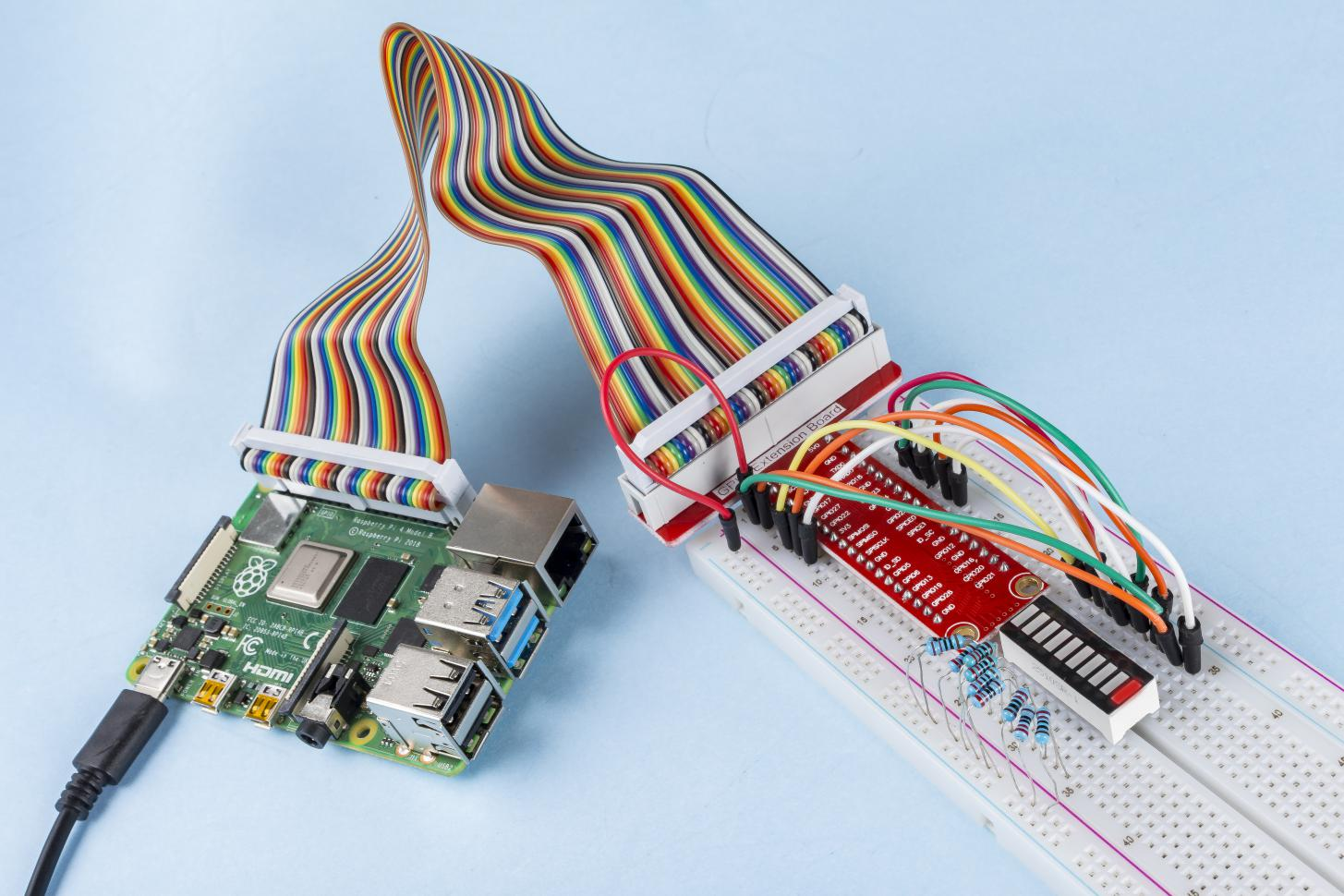# 1.1.3 LED Bar Graph¶

## Introduction¶

In this project, we sequentially illuminate the lights on the LED Bar Graph.

## Components¶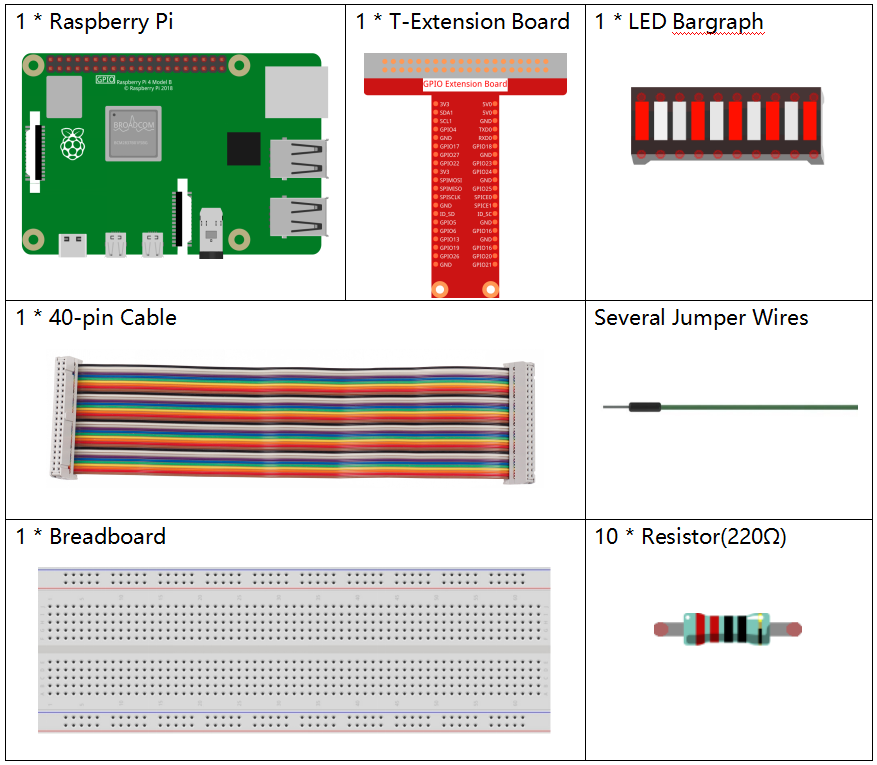## Principle¶

LED Bar Graph

LED Bar Graph is an LED array, which is used to connect with electronic circuit or microcontroller. It’s easy to connect LED bar graph with the circuit like as connecting 10 individual LEDs with 10 output pins. Generally we can use the LED bar graph as a Battery level Indicator, Audio equipments, and Industrial Control panels. There are many other applications of LED bar graphs.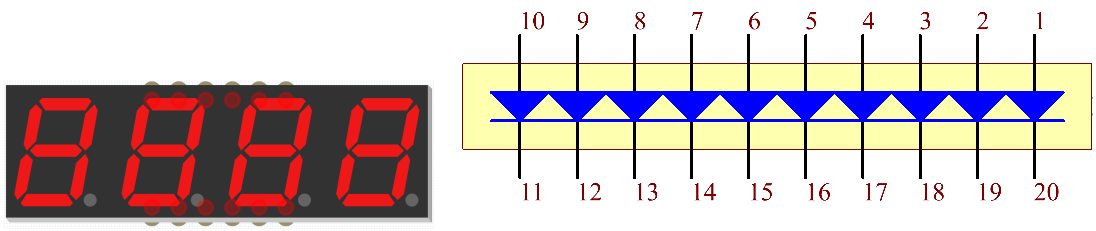## Schematic Diagram¶

 T-Board Name physical wiringPi BCM GPIO17 Pin 11 0 17 GPIO18 Pin 12 1 18 GPIO27 Pin 13 2 27 GPIO22 Pin 15 3 22 GPIO23 Pin 16 4 23 GPIO24 Pin 18 5 24 GPIO25 Pin 22 6 25 SDA1 Pin 3 8 2 SCL1 Pin 5 9 3 SPICE0 Pin 24 10 8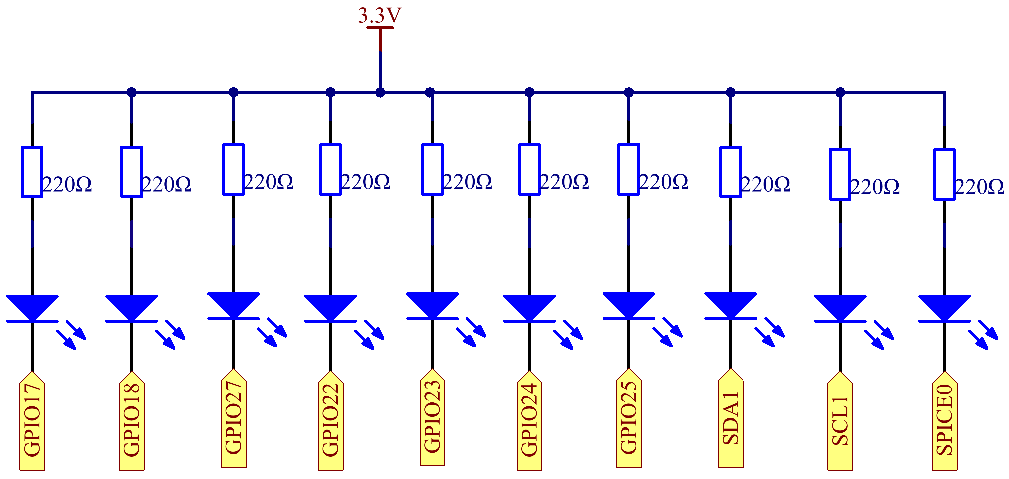## Experimental Procedures¶

Step 1: Build the circuit.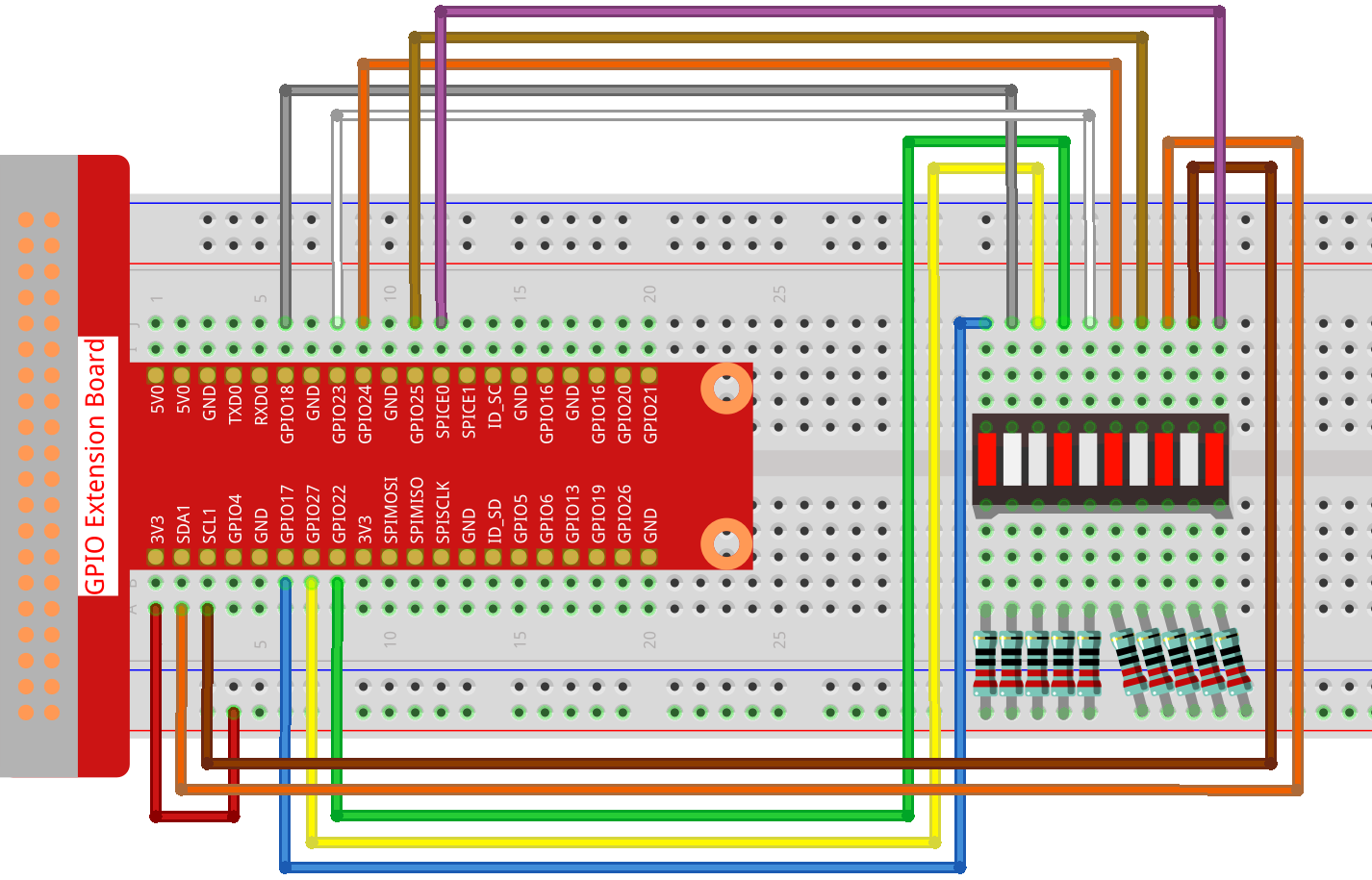### For C Language Users¶

Step 2: Go to the folder of the code.

```cd ~/davinci-kit-for-raspberry-pi/c/1.1.3/
```

Step 3: Compile the code.

```gcc 1.1.3_LedBarGraph.c -lwiringPi
```

Step 4: Run the executable file.

```sudo ./a.out
```

After the code runs, you will see the LEDs on the LED bar turn on and off regularly.

Note

If it does not work after running, or there is an error prompt: “wiringPi.h: No such file or directory”, please refer to C code is not working?.

Code

```#include <wiringPi.h>
#include <stdio.h>

int pins = {0,1,2,3,4,5,6,8,9,10};
void oddLedBarGraph(void){
for(int i=0;i<5;i++){
int j=i*2;
digitalWrite(pins[j],HIGH);
delay(300);
digitalWrite(pins[j],LOW);
}
}
void evenLedBarGraph(void){
for(int i=0;i<5;i++){
int j=i*2+1;
digitalWrite(pins[j],HIGH);
delay(300);
digitalWrite(pins[j],LOW);
}
}
void allLedBarGraph(void){
for(int i=0;i<10;i++){
digitalWrite(pins[i],HIGH);
delay(300);
digitalWrite(pins[i],LOW);
}
}
int main(void)
{
if(wiringPiSetup() == -1){ //when initialize wiring failed,print message to screen
printf("setup wiringPi failed !");
return 1;
}
for(int i=0;i<10;i++){       //make led pins' mode is output
pinMode(pins[i], OUTPUT);
digitalWrite(pins[i],LOW);
}
while(1){
oddLedBarGraph();
delay(300);
evenLedBarGraph();
delay(300);
allLedBarGraph();
delay(300);
}
return 0;
}
```

Code Explanation

```int pins = {0,1,2,3,4,5,6,8,9,10};
```

Create an array and assign it to the pin number corresponding to the LED Bar Graph (0,1,2,3,4,5,6,8,9,10) and the array will be used to control the LED.

```void oddLedBarGraph(void){
for(int i=0;i<5;i++){
int j=i*2;
digitalWrite(pins[j],HIGH);
delay(300);
digitalWrite(pins[j],LOW);
}
}
```

Let the LED on the odd digit of the LED Bar Graph light on in turn.

```void evenLedBarGraph(void){
for(int i=0;i<5;i++){
int j=i*2+1;
digitalWrite(pins[j],HIGH);
delay(300);
digitalWrite(pins[j],LOW);
}
}
```

Make the LED on the even digit of the LED Bar Graph light on in turn.

```void allLedBarGraph(void){
for(int i=0;i<10;i++){
digitalWrite(pins[i],HIGH);
delay(300);
digitalWrite(pins[i],LOW);
}
}
```

Let the LED on the LED Bar Graph light on one by one.

### For Python Language Users¶

Step 2: Go to the folder of the code.

```cd /home/pi/davinci-kit-for-raspberry-pi/python/
```

Step 3: Run the executable file.

```sudo python3 1.1.3_LedBarGraph.py
```

After the code runs, you will see the LEDs on the LED bar turn on and off regularly.

Code

Note

You can Modify/Reset/Copy/Run/Stop the code below. But before that, you need to go to source code path like `davinci-kit-for-raspberry-pi/python`.

```import RPi.GPIO as GPIO
import time

ledPins = [11, 12, 13, 15, 16, 18, 22, 3, 5, 24]

def oddLedBarGraph():
for i in range(5):
j = i*2
GPIO.output(ledPins[j],GPIO.HIGH)
time.sleep(0.3)
GPIO.output(ledPins[j],GPIO.LOW)

def evenLedBarGraph():
for i in range(5):
j = i*2+1
GPIO.output(ledPins[j],GPIO.HIGH)
time.sleep(0.3)
GPIO.output(ledPins[j],GPIO.LOW)

def allLedBarGraph():
for i in ledPins:
GPIO.output(i,GPIO.HIGH)
time.sleep(0.3)
GPIO.output(i,GPIO.LOW)

def setup():
GPIO.setwarnings(False)
GPIO.setmode(GPIO.BOARD)        # Numbers GPIOs by physical location
for i in ledPins:
GPIO.setup(i, GPIO.OUT)   # Set all ledPins' mode is output
GPIO.output(i, GPIO.LOW) # Set all ledPins to high(+3.3V) to off led

def loop():
while True:
oddLedBarGraph()
time.sleep(0.3)
evenLedBarGraph()
time.sleep(0.3)
allLedBarGraph()
time.sleep(0.3)

def destroy():
for pin in ledPins:
GPIO.output(pin, GPIO.LOW)    # turn off all leds
GPIO.cleanup()                     # Release resource

if __name__ == '__main__':     # Program start from here
setup()
try:
loop()
except KeyboardInterrupt:  # When 'Ctrl+C' is pressed, the program destroy() will be  executed.
destroy()
```

Code Explanation

ledPins = [11, 12, 13, 15, 16, 18, 22, 3, 5, 24] Create an array and assign it to the pin number corresponding to the LED Bar Graph (11, 12, 13, 15, 16, 18, 22, 3, 5, 24) and the array will be used to control the LED.

```def oddLedBarGraph():
for i in range(5):
j = i*2
GPIO.output(ledPins[j],GPIO.HIGH)
time.sleep(0.3)
GPIO.output(ledPins[j],GPIO.LOW)
```

Let the LED on the odd digit of the LED Bar Graph light on in turn.

```def evenLedBarGraph():
for i in range(5):
j = i*2+1
GPIO.output(ledPins[j],GPIO.HIGH)
time.sleep(0.3)
GPIO.output(ledPins[j],GPIO.LOW)
```

Make the LED on the even digit of the LED Bar Graph light on in turn.

```def allLedBarGraph():
for i in ledPins:
GPIO.output(i,GPIO.HIGH)
time.sleep(0.3)
GPIO.output(i,GPIO.LOW)
```

Let the LED on the LED Bar Graph light on one by one.

## Phenomenon Picture¶